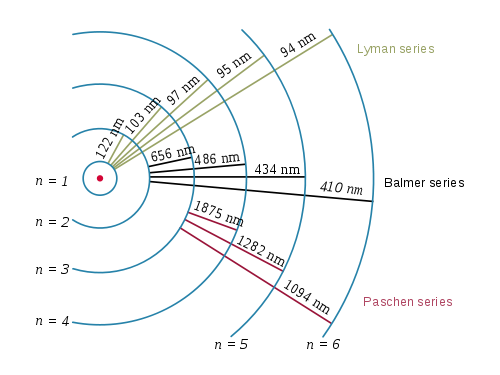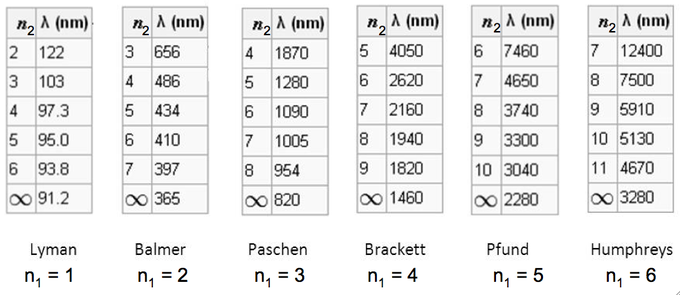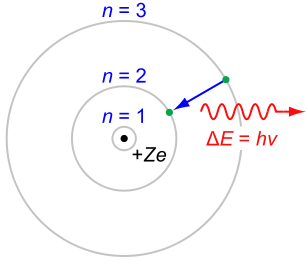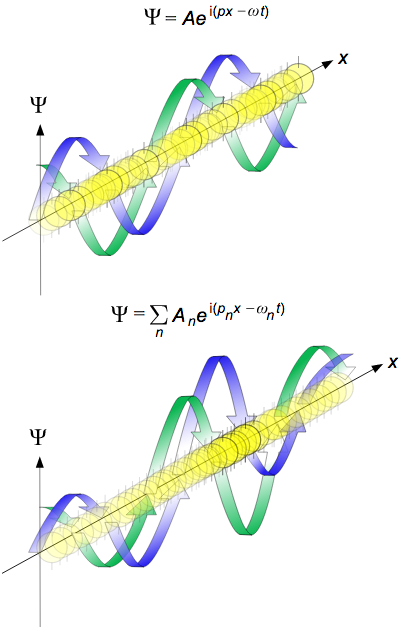## Emission Spectrum of the Hydrogen Atom

The emission spectrum of atomic hydrogen is divided into a number of spectral series.

### Learning Objectives

Calculate emission spectra for Hydrogen using the Rydberg formula

### Key Takeaways

#### Key Points

• The wavelengths in a spectral series are given by the Rydberg formula.
• The observed spectral lines are due to electrons moving between energy levels in an atom.
• Further series are unnamed but follow exactly the same pattern as dictated by the Rydberg equation.

#### Key Terms

• spectrum: A range of colors representing light (electromagnetic radiation) of contiguous frequencies; hence electromagnetic spectrum, visible spectrum, ultraviolet spectrum, etc.
• emission: In a spectral sense, what occurs when an electron transitions between a higher energy level and a lower one, resulting in the release of a photon of predictable energy.

The emission spectrum of a chemical element or chemical compound is the spectrum of frequencies of electromagnetic radiation emitted by an atom’s electrons when they are returned to a lower energy state. Each element’s emission spectrum is unique, and therefore spectroscopy can be used to identify elements present in matter of unknown composition. Similarly, the emission spectra of molecules can be used in chemical analysis of substances.The spectral series of hydrogen, on a logarithmic scale

The emission spectrum of atomic hydrogen is divided into a number of spectral series, with wavelengths given by the Rydberg formula: $\frac { 1 }{ \lambda_{vac} } =RZ^2( \frac { 1 }{ {n_1 }^{ 2 } } -\frac { 1 }{ { n_2 }^{ 2 } })$,

where R is the Rydberg constant (approximately 1.09737 x 107 m-1), $\lambda_{vac}$ is the wavelength of the light emitted in vacuum, Z is the atomic number, and n1 and n2 are integers representing the energy levels involved such that n1 < n2. All observed spectral lines are due to electrons moving between energy levels in the atom. The spectral series are important in astronomy for detecting the presence of hydrogen and calculating red shifts. Further series for hydrogen as well as other elements were discovered as spectroscopy techniques developed.

The line spectrum of hydrogen: Explain how the lines in the emission spectrum of hydrogen are related to electron energy levels. You need to understand convergence, production of UV, vis, IR, excitation, concentric energy levels and be able to draw the line spectra.

The spectral lines are grouped into series according to n′. Lines are named sequentially starting from the longest wavelength/lowest frequency of the series using Greek letters within each series. For example, the 2 → 1 line is called Lyman-alpha (Ly-α), while the 7 → 3 line is called Paschen-delta (Pa-δ). Some hydrogen spectral lines fall outside these series, such as the 21 cm line (these correspond to much rarer atomic events such as hyperfine transitions). The fine structure also results in single spectral lines appearing as two or more closely grouped thinner lines due to relativistic corrections. Typically, one can only observe these series from pure hydrogen samples in a lab. Many of the lines are very faint and additional lines can be caused by other elements (such as helium if using sunlight or nitrogen in the air). Lines outside of the visible spectrum typically cannot be seen in observations of sunlight, as the atmosphere absorbs most infrared and ultraviolet wavelengths through the action of water vapor and ozone molecules respectively.Electron transitions and their resulting wavelengths for hydrogen: Three of the transition series in hydrogen (not to scale).The emission spectrum of hydrogen: Some of the most common and readily observable series have been named as shown in this image, where n1 is the ground state and n2 are excited states. The various series are named for the atomic energy level they end on (n1). The series limit where n2 is infinite and n1=1 corresponds to the ionization energy of hydrogen.

## The Bohr Model

The Bohr model depicts atoms as small, positively charged nuclei surrounded by electrons in circular orbits.

### Learning Objectives

Explain how the Bohr model of the atom marked an improvement over earlier models, but still had limitations from its use of Maxwell’s theory

### Key Takeaways

#### Key Points

• The model’s success lay in explaining the Rydberg formula for the spectral emission lines of atomic hydrogen.
• The model states that electrons in atoms move in circular orbits around a central nucleus and can only orbit stably in certain fixed circular orbits at a discrete set of distances from the nucleus. These orbits are associated with definite energies and are also called energy shells or energy levels.
• In these stable orbits, an electron’s acceleration does not result in radiation and energy loss as required by classical electromagnetic theory.

#### Key Terms

• correspondence principle: States that the behavior of systems described by the theory of quantum mechanics (or by the old quantum theory) reproduces classical physics in the limit of large quantum number.
• unstable: For an electron orbiting the nucleus, according to classical mechanics, it would mean an orbit of decreasing radius and approaching the nucleus in a spiral trajectory.
• emission: Act of releasing or giving away, energy in the case of the electron.

In atomic physics, the Bohr model depicts an atom as a small, positively charged nucleus surrounded by electrons. These electrons travel in circular orbits around the nucleus—similar in structure to the solar system, except electrostatic forces rather than gravity provide attraction.

The Rutherford–Bohr model of the hydrogen atom. In this view, electron orbits around the nucleus resemble that of planets around the sun in the solar system.The Bohr atom: The Rutherford-Bohr model of the hydrogen atom (= 1) or a hydrogen-like ion (> 1), where the negatively charged electron confined to an atomic shell encircles a small, positively charged atomic nucleus and where an electron jump between orbits is accompanied by an emitted or absorbed amount of electromagnetic energy (hv). The orbits in which the electron may travel are shown as grey circles; their radius increases as n2, where n is the principal quantum number. The 3 → 2 transition depicted here produces the first line of the Balmer series, and for hydrogen (Z = 1), it results in a photon wavelength 656 nm (red light).

### Development of the Bohr Model

The Bohr model was an improvement on the earlier cubic model (1902), the plum-pudding model (1904), the Saturnian model (1904), and the Rutherford model (1911). Since the Bohr model is a quantum -physics-based modification of the Rutherford model, many sources combine the two: the Rutherford–Bohr model.

Although it challenged the knowledge of classical physics, the model’s success lay in explaining the Rydberg formula for the spectral emission lines of atomic hydrogen. While the Rydberg formula had been known experimentally, it did not gain a theoretical underpinning until the Bohr model was introduced. Not only did the Bohr model explain the reason for the structure of the Rydberg formula, it also provided a justification for its empirical results in terms of fundamental physical constants.

Although revolutionary at the time, the Bohr model is a relatively primitive model of the hydrogen atom compared to the valence shell atom. As an initial hypothesis, it was derived as a first-order approximation to describe the hydrogen atom. Due to its simplicity and correct results for selected systems, the Bohr model is still commonly taught to introduce students to quantum mechanics. A related model, proposed by Arthur Erich Haas in 1910, was rejected. The quantum theory from the period between Planck’s discovery of the quantum (1900) and the advent of a full-blown quantum mechanics (1925) is often referred to as the old quantum theory.

Early planetary models of the atom suffered from a flaw: they had electrons spinning in orbit around a nucleus—a charged particle in an electric field. There was no accounting for the fact that the electron would spiral into the nucleus. In terms of electron emission, this would represent a continuum of frequencies being emitted since, as the electron moved closer to the nucleus, it would move faster and would emit a different frequency than those experimentally observed. These planetary models ultimately predicted all atoms to be unstable due to the orbital decay. The Bohr theory solved this problem and correctly explained the experimentally obtained Rydberg formula for emission lines.

### Properties of Electrons under the Bohr Model

In 1913, Bohr suggested that electrons could only have certain classical motions:

1. Electrons in atoms orbit the nucleus.
2. The electrons can only orbit stably, without radiating, in certain orbits (called by Bohr the “stationary orbits”) at a certain discrete set of distances from the nucleus. These orbits are associated with definite energies and are also called energy shells or energy levels. In these orbits, an electron’s acceleration does not result in radiation and energy loss as required by classical electromagnetic theory.
3. Electrons can only gain or lose energy by jumping from one allowed orbit to another, absorbing or emitting electromagnetic radiation with a frequency (ν) determined by the energy difference of the levels according to the Planck relation.

Behavior of Electrons: Part 3, The Bohr Model of the Atom – YouTube: We combine our new found knowledge of the nature of light with Bohr’s atomic theory.

Bohr’s model is significant because the laws of classical mechanics apply to the motion of the electron about the nucleus only when restricted by a quantum rule. Although Rule 3 is not completely well defined for small orbits, Bohr could determine the energy spacing between levels using Rule 3 and come to an exactly correct quantum rule—the angular momentum L is restricted to be an integer multiple of a fixed unit:

$L=n\frac { h }{ 2\pi } =n\hbar$

where n = 1, 2, 3,… is called the principal quantum number and ħ = h/2π. The lowest value of n is 1; this gives a smallest possible orbital radius of 0.0529 nm, known as the Bohr radius. Once an electron is in this lowest orbit, it can get no closer to the proton. Starting from the angular momentum quantum rule, Bohr was able to calculate the energies of the allowed orbits of the hydrogen atom and other hydrogen-like atoms and ions.

### The Correspondence Principle

Like Einstein’s theory of the photoelectric effect, Bohr’s formula assumes that during a quantum jump, a discrete amount of energy is radiated. However, unlike Einstein, Bohr stuck to the classical Maxwell theory of the electromagnetic field. Quantization of the electromagnetic field was explained by the discreteness of the atomic energy levels. Bohr did not believe in the existence of photons.

According to the Maxwell theory, the frequency (ν) of classical radiation is equal to the rotation frequency (νrot) of the electron in its orbit, with harmonics at integer multiples of this frequency. This result is obtained from the Bohr model for jumps between energy levels En and En−k when k is much smaller than n. These jumps reproduce the frequency of the k-th harmonic of orbit n. For sufficiently large values of n (so-called Rydberg states), the two orbits involved in the emission process have nearly the same rotation frequency so that the classical orbital frequency is not ambiguous. But for small n (or large k), the radiation frequency has no unambiguous classical interpretation. This marks the birth of the correspondence principle, requiring quantum theory to agree with the classical theory only in the limit of large quantum numbers.

The Bohr-Kramers-Slater theory (BKS theory) is a failed attempt to extend the Bohr model, which violates the conservation of energy and momentum in quantum jumps, with the conservation laws only holding on average.

## The de Broglie Wavelength

The de Broglie wavelength is inversely proportional to the momentum of a particle.

### Learning Objectives

Use the de Broglie equations to determine the wavelength, momentum, frequency, or kinetic energy of particles

### Key Takeaways

#### Key Points

• At the end of the 19th century, light was thought to consist of waves of electromagnetic fields that propagated according to Maxwell’s equations, while matter was thought to consist of localized particles.
• In his 1905 paper on the photoelectric effect, Albert Einstein postulated that light was emitted and absorbed as localized packets or quanta (now called photons ).
• De Broglie showed that the theory of relativity was applicable to not just photons but also all particles with linear momentum, and therefore that matter, including electrons, also has wave-like properties.

#### Key Terms

• wavelength: The distance between one peak or trough of a travelling oscillation and the next; it is often designated in physics as λ and corresponds to the velocity divided by the frequency.
• frequency: The number of occurrences of a repeating event per unit of time.

At the end of the 19th century, light was thought to consist of waves of electromagnetic fields that propagated according to Maxwell’s equations, while matter was thought to consist of localized particles. This division was challenged when, in his 1905 paper on the photoelectric effect, Albert Einstein postulated that light was emitted and absorbed as localized packets or quanta (now called photons). These quanta would have an energy of:

$E=hv$

where v is the frequency of the light and h is Planck’s constant. Einstein’s postulate was confirmed experimentally by Robert Millikan and Arthur Compton over the next two decades. Thus it became apparent that light has both wave-like and particle-like properties. In his 1924 PhD thesis, de Broglie sought to expand this wave-particle duality to all material particles with linear momentum.de Broglie waves: Propagation of de Broglie waves in 1 dimension (the real part of the complex amplitude is blue and the imaginary part is green; top: plane wave, bottom: wave packet.). The probability (shown as the color opacity) of finding the particle at a given point x is spread out like a waveform, with no definite position of the particle. As the amplitude increases above zero the curvature decreases, so the amplitude decreases again, and vice versa – the result is an alternating amplitude: a wave.

In 1926, Erwin Schrödinger published an equation describing how a matter wave should evolve—the matter wave equivalent of Maxwell’s equations—and used it to derive the energy spectrum of hydrogen. That same year, Max Born published his now-standard interpretation that the square of the amplitude of a matter wave gives the probability of finding a particle at a given place. This interpretation was in contrast to de Broglie’s own interpretation, in which the wave corresponds to the physical motion of a localized particle.

### The De Broglie Equations

The de Broglie equations relate the wavelength (λ) to the momentum (p), and the frequency (f) to the kinetic energy (E) (excluding its rest energy and any potential energy) of a particle:

$\lambda ={ h }/p$ and $f={ E }/{ h }$

where h is Planck’s Constant. The two equations can be equivalently written as

$p =\hbar k$ and $E=\hbar \omega$

where $\hbar ={ h }/{ 2\pi }$ is the reduced Planck’s constant,

where $k={ 2\pi }/{ \lambda }$ is the angular wavenumber, and

where $\omega =2\pi f$ is the angular frequency.

De Broglie’s key realization was that in a one-electron atom, for a wave to have a stable amplitude and not decay over time, an integer number (n) of wavelengths must fit into a single circumference drawn by the Bohr orbit. He related this to the principal quantum number n through the equation:

$n\lambda = 2\pi{r}$

This was fortunately reminiscent of Bohr’s observation about the angular momentum of an electron, which had already been established:

$m_{e}vr=2\pi{r}$

By inspection of the above and with minor rearrangement, the de Broglie relation can be obtained as follows:

$\lambda = \frac{h}{m_{e}{v}} = \frac{h}{p}$

Doc Physics – de Broglie: I include a summary of the hydrogen atom’s electronic structure and explain how an electron can interfere with itself in an orbit just like it can in a double-slit experiment.

### Confirming the de Broglie Hypothesis

In 1927 at Bell Labs, Clinton Davisson and Lester H. Germer fired slow-moving electrons at a crystalline nickel target. The angular dependence of the reflected electron intensity was measured and was determined to have the same diffraction pattern as those predicted by Bragg for X-rays. Before the acceptance of the de Broglie hypothesis, diffraction was a property that was thought to only be exhibited by waves. Therefore, the presence of any diffraction effects by matter demonstrated the wave-like nature of matter. When the de Broglie wavelength was inserted into the Bragg condition, the observed diffraction pattern was predicted, thereby experimentally confirming the de Broglie hypothesis for electrons.

Experiments with Fresnel diffraction and specular reflection of neutral atoms confirm the application of the de Broglie hypothesis to atoms, i.e. the existence of atomic waves that undergo diffraction, interference, and allow quantum reflection by the tails of the attractive potential. Advances in laser cooling have allowed the cooling down of neutral atoms to temperatures near absolute zero. At these temperatures, the thermal de Broglie wavelengths come into the micrometer range. Using Bragg diffraction of atoms and a Ramsey interferometry technique, the de Broglie wavelength of cold sodium atoms was explicitly measured and found to be consistent with the temperature measured by a different method.

Recent experiments even confirm the de Broglie relations for molecules and macromolecules, which are normally considered too large to undergo quantum mechanical effects. In 1999, a research team in Vienna demonstrated diffraction for molecules as large as fullerenes. The researchers calculated a de Broglie wavelength of the most probable C60 velocity as 2.5 pm. More recent experiments prove the quantum nature of molecules with a mass up to 6910 amu. In general, the de Broglie hypothesis is expected to apply to any well-isolated object. Even macroscopic objects like tennis balls have a calculable de Broglie wavelength; however, they would be much too small to observe experimentally, and their wave-like nature is not intuitive to common experience.

## The Uncertainty Principle

Only partial knowledge of the momentum and position of a particle may be known at the same time.

### Learning Objectives

Summarize the uncertainty principle of quantum mechanics.

### Key Takeaways

#### Key Points

• The uncertainty principle is written as ${ \sigma }_{ x }{ \sigma }_{\rho }\quad\ge\quad\frac{ \hbar }{ 2 }$.
• The position of an object cannot be known simultaneously with its momentum.
• The more precisely one quantity is known, the less precisely the other is known.

#### Key Terms

• momentum: The product of the mass and velocity of a particle in motion.
• uncertainty: A parameter that measures the dispersion of a range of measured values.

In quantum mechanics, the uncertainty principle is any of a variety of mathematical inequalities asserting a fundamental limit to the precision with which certain pairs of physical properties of a particle, such as position (x) and momentum (p), can be known simultaneously. The more precisely the position of some particle is determined, the less precisely its momentum can be known, and vice versa. The original heuristic argument that such a limit should exist was given by Werner Heisenberg in 1927, after whom it is sometimes named as the Heisenberg Uncertainty Principle. The reasoning was derived from considering the uncertainty in both the position and the momentum of an object. Roughly, the uncertainty in the position of a particle is approximately equal to its wavelength (λ). The uncertainty in the momentum of the object follows from de Broglie’s equation as h/λ. Therefore, to a first approximation the Heisenberg Uncertainty Principle gives that the product of these two uncertainties is on the order of Planck’s constant (h).

A more formal inequality relating the standard deviation of position (${ \sigma }_{ x }$) and the standard deviation of momentum (${ \sigma }_{ \rho }$) was derived by Earle Hesse Kennard later that year (and independently by Hermann Weyl in 1928):

${ \sigma }_{ x }{ \sigma }_{\rho }\quad\ge\quad\frac{ \hbar }{ 2 }$

Historically, the uncertainty principle has been confused with a somewhat similar effect in physics, called the observer effect, which notes that measurements of certain systems cannot be made without affecting the systems. Heisenberg offered such an observer effect at the quantum level as a physical explanation of quantum uncertainty. It has since become clear, however, that the uncertainty principle is inherent in the properties of all wave-like systems and that it arises in quantum mechanics simply due to the matter-wave nature of all quantum objects.

Doc Physics – Heisenberg Uncertainty Principle Derived and Explained – YouTube: One of the most-oft quoted results of quantum physics, this doozie forces us to reconsider what we can know about the universe. Some things cannot be known simultaneously. In fact, if anything about a system is known perfectly, there is likely another characteristic that is completely shrouded in uncertainty. So significant figures ARE important after all!

### Scope of the Uncertainty Principle and Applications

The uncertainty principle actually states a fundamental property of quantum systems and is not a statement about the observational success of current technology. It must be emphasized that measurement does not mean only a process in which a physicist-observer takes part, but rather any interaction between classical and quantum objects regardless of any observer. Since the uncertainty principle is such a basic result in quantum mechanics, typical experiments in quantum mechanics routinely observe aspects of it. Certain experiments, however, may deliberately test a particular form of the uncertainty principle as part of their main research program. These include, for example, tests of number-phase uncertainty relations in superconducting or quantum optics systems. Applications are for developing extremely low noise technology, such as that required in gravitational-wave interferometers.